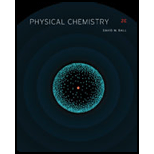# (a) If the electron were to have a positive charge, what would be the difference in the magnitude calculated in Example 16. 2b for the magnetic dipole caused by an electron in the hydrogen atom? (b) Positronium is very similar to a hydrogen atom except that instead of a proton in the nucleus, there is a positron. (This short-lived “element” can be made in the laboratory.) On the basis of your answer to part a, what is the overall magnetic dipole caused by the two particles in mutual orbit about each other?### Physical Chemistry

2nd Edition
Ball + 3 others
ISBN: 9781133958437

#### Solutions

Chapter
Section### Physical Chemistry

2nd Edition
Ball + 3 others
ISBN: 9781133958437
Chapter 16, Problem 16.3E
Textbook Problem
1 views

## (a) If the electron were to have a positive charge, what would be the difference in the magnitude calculated in Example 16. 2b for the magnetic dipole caused by an electron in the hydrogen atom?(b) Positronium is very similar to a hydrogen atom except that instead of a proton in the nucleus, there is a positron. (This short-lived “element” can be made in the laboratory.) On the basis of your answer to part a, what is the overall magnetic dipole caused by the two particles in mutual orbit about each other?

Interpretation Introduction

(a)

Interpretation:

The difference in the magnitude calculated in Example 16.2b for the magnetic dipole caused by an electron in the hydrogen atom when the electron has a positive charge is to be stated.

Concept introduction:

The dipole moment is defined as the charge separation between two atoms or ions. It is used to determine the polarity of atoms or ions.

The magnetic dipole, μ is calculated by the formula shown below.

μ=I×A

### Explanation of Solution

The magnetic dipole, μ in Example 16.2b is calculated by the formula shown below.

μ=I×Aμ=I×πr2

Interpretation Introduction

(b)

Interpretation:

The overall magnetic dipole caused by the two particles in mutual orbit about each other is to be calculated.

Concept introduction:

The dipole moment is defined as the charge separation between two atoms or ions. It is used to determine the polarity of atoms or ions.

The magnetic dipole, μ is calculated by the formula shown below.

μ=I×A

### Still sussing out bartleby?

Check out a sample textbook solution.

See a sample solution

#### The Solution to Your Study Problems

Bartleby provides explanations to thousands of textbook problems written by our experts, many with advanced degrees!

Get Started

Find more solutions based on key concepts
Vitamin A supplements can help treat acne. T F

Nutrition: Concepts and Controversies - Standalone book (MindTap Course List)

Where would you go on Earth to have a celestial pole at your zenith?

Horizons: Exploring the Universe (MindTap Course List)

What happens to the energy of light when light is absorbed in seawater?

Oceanography: An Invitation To Marine Science, Loose-leaf Versin

A(n) ________ substance repels water. a. acidic b. basic c. hydrophobic d. polar

Biology: The Unity and Diversity of Life (MindTap Course List)

A wind turbine on a wind farm turns in response to a force of high-speed air resistance, R=12DAv2. The power av...

Physics for Scientists and Engineers, Technology Update (No access codes included)